# Data comparison instruction:

PLC instructions are designed to receive and manipulate non-Boolean values such as these to perform useful control functions. The IEC 61131-3 standard specifies a variety of data comparison instructions for comparing two non-Boolean values, and generating Boolean outputs.

The Q output for each instruction “box” activates whenever the evaluated comparison function is “true” and the enable input (EN) is active. If the enable input remains active but the comparison function is false, the Q output de-activates. If the enable input de-de-activates, the Q output retains its last state.

Equal: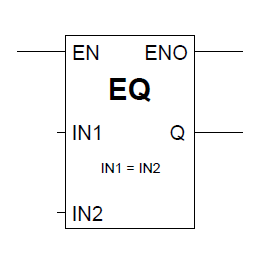Not equal: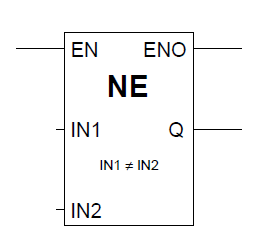Greater than (>):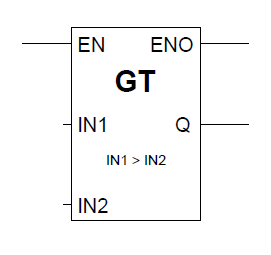Less than (<):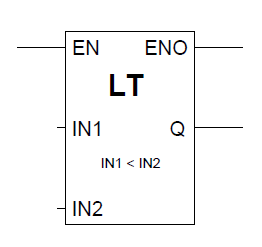Greater than or equal to(≥) :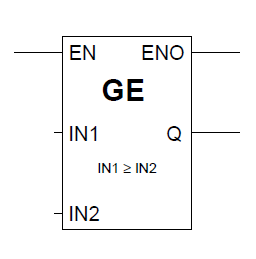Less than or equal to (≤):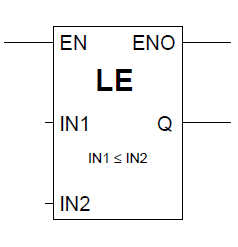# Math instructions:

The IEC 61131-3 standard specifies several dedicated ladder instructions for performing arithmetic calculations.

As with the data comparison instructions, each of these math instructions must be enabled by an “energized” signal to the enable (EN) input. Input and output values are linked to each math instruction by tag name.

There are two types of math instructions, two input and one input.

Two input instructions:

Addition, Substraction, Percentage, Multiplication, Division, Exponential.

One input instruction:

Function of input is given as the output.

SIN, COS, TAN, LN, LOG, Exeponential, Square root,